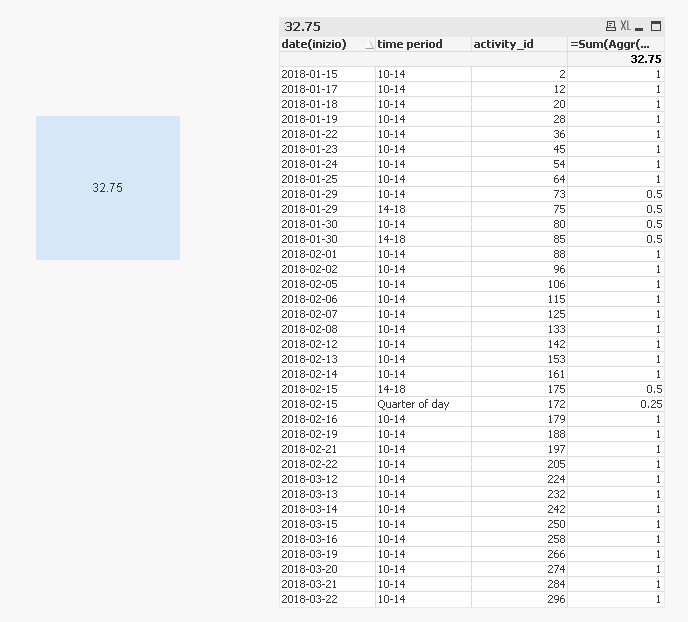# New to QlikView

Discussion board where members can get started with QlikView.

Contributor II

## Calculate percentage of occupation of the day

Assuming I have the following table:

activity_idDateactivity_typetime periodPercentage of occupation
12018-01-168whole day 100
22018-01-17610-1425
32018-01-18610-1425
42018-01-18814-1825
52018-01-19710-1425
62018-01-19614-1825

I have to calculate how much percentage did the activity_type = 6 occupy for all the different dates with the following condition:
If there's a day in which only activities of type 6 happened, the calculated percentage should be 100%
Case in point the expected result should be 37.5%( on date 2018-01-17 there's only a single type of activity, so the calculated percentage is 100%; 100% + 25% from activity_id = 3 + 25% from activity_id = 6 = 150% divided by the number of days: 4)

Tags (1)
1 Solution

Accepted SolutionsMVP

## Re: Calculate percentage of occupation of the day

Try this

=Sum(Aggr(If(RowNo() = 1, Sum({<activity_type = {'6'}, [date(inizio)] = {"=Count(DISTINCT activity_type) > 1"}>} [Percentage of occupation])), [date(inizio)], [time period], activity_id)) +

Sum(Aggr(If(RowNo() = 1, Sum({<activity_type = {'6'}, [date(inizio)] = {"=Count(DISTINCT activity_type) = 1"}>} 1)), [date(inizio)], activity_id))25 RepliesMVP

## Re: Calculate percentage of occupation of the day

May be this

Sum({<activity_type = {'6'}>} [Percentage of occupation])/Count(DISTINCT Date)

Contributor II

## Re: Calculate percentage of occupation of the day

normally this would work, but how should I tackle the case in which a single day has only activities of activity_type 2? Case in point, for activity_id = 2, because it's the only activity for that day, the value should be 100 when I calculate the expressionMVP

## Re: Calculate percentage of occupation of the day

You mean activity 6, right?... checking

Contributor II

## Re: Calculate percentage of occupation of the day

activity 6 is on the same day as activity 5, so its percentage of occupation should be unchangedMVP

## Re: Calculate percentage of occupation of the day

Try this

=(Sum({<activity_type = {'6'}, Date = {"=Count(DISTINCT activity_type) > 1"}>} [Percentage of occupation]) + Sum({<activity_type = {'6'}, Date = {"=Count(DISTINCT activity_type) = 1"}>} 100))/Count(DISTINCT Date)MVP

## Re: Calculate percentage of occupation of the day

My bad, I got confused between activity and activity_type... I thought you were talking about activity_type...

Contributor II

## Re: Calculate percentage of occupation of the day

Almost there. How can I add a filter to take only the activities after a certain Date in a set expression?MVP

## Re: Calculate percentage of occupation of the day

May be like this

=(Sum({<activity_type = {'6'}, Date = {"=Count(DISTINCT activity_type) > 1"}*{YourDateRange}>} [Percentage of occupation]) + Sum({<activity_type = {'6'}, Date = {"=Count(DISTINCT activity_type) = 1"}*{YourDateRange}>} 100))/Count({<Date = {YourDateRange}>} DISTINCT Date)

Contributor II

## Re: Calculate percentage of occupation of the day

Could you give me an example for how to use {YourDateRange}?